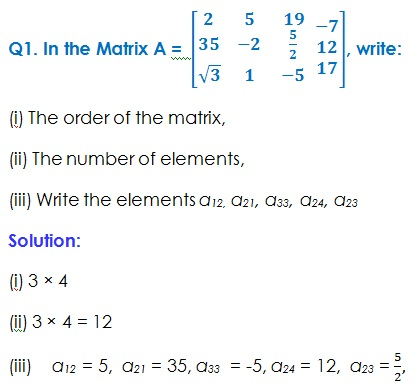Study Materials

# NCERT Solutions for Class 12th Mathematics-I

Page 1 of 5

## Chapter 3. Matrices

### Exercise 3.1

Exercise-3.1Q2.  If a matrix has 24 elements, what are the possible orders it can have? What, if it has 13 elements?

Solution:

(i) The possible order of Matrix will be followings

24 × 1, 1 × 24, 12 × 2,  2 × 12,  6 × 4, 4 ×6, 8 × 3, 3 × 8

No of orders = 8.

(ii) Matrix of elements 13 having the order 1 × 13, 13 × 1

No of orders  = 2

Q3. If a matrix has 18 elements, what are the possible orders it can have? What, if it has 5 elements?

Solution:

Possible Order for Matrix of having elements 18,

1 × 18, 18 × 1, 9 × 2, 2 × 9, 6 × 3, 3 ×6

No of order = 6

Page 1 of 5

Chapter Contents: